Ohio EOCE Algebra I: Quadratic Equations Chapter Exam

Exam Instructions:

Choose your answers to the questions and click 'Next' to see the next set of questions. You can skip questions if you would like and come back to them later with the yellow "Go To First Skipped Question" button. When you have completed the practice exam, a green submit button will appear. Click it to see your results. Good luck!

Answered 0 of 30

Page 1

Question 1 1. In order to complete the square on the following equation, what value would you add to both sides?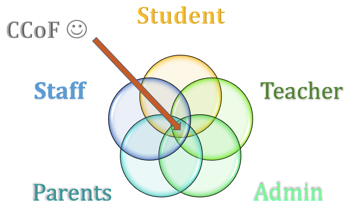Page 2

Question 7 7. Rewrite the following equation in the vertex form.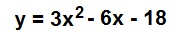Question 10 10. What would the first step be in completing the square of the following equation?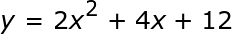Page 4

Question 16 16. Solve the equation below.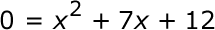Question 17 17. When completing the square on the quadratic equation listed below, how is c calculated?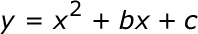Question 20 20. Find the value for c and rewrite the equation in the vertex form.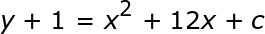Page 5

Question 21 21. Rewrite the quadratic below in standard form: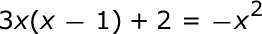Question 23 23. Find the roots of the equation.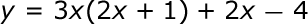Question 25 25. Solve the equation below.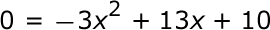Page 6

Question 26 26. Expressing the equation below in the vertex form will give a value of 'h' = _____ .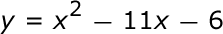Ohio EOCE Algebra I: Quadratic Equations Chapter Exam Instructions

Choose your answers to the questions and click 'Next' to see the next set of questions. You can skip questions if you would like and come back to them later with the yellow "Go To First Skipped Question" button. When you have completed the practice exam, a green submit button will appear. Click it to see your results. Good luck!

Support